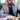# Leetcode 1028 Recover a Tree From Preorder Traversal

January 02, 2021

### Problem statement

We run a preorder depth-first search (DFS) on the root of a binary tree.

At each node in this traversal, we output D dashes (where D is the depth of this node), then we output the value of this node. If the depth of a node is D, the depth of its immediate child is D + 1. The depth of the root node is 0.

If a node has only one child, that child is guaranteed to be the left child.

Given the output S of this traversal, recover the tree and return its root.

### Intuition

We modify the original preorder traversal template to solve this problem. Preorder traversal looks like the following

``````def preorder(node):
if not node: return
process(node)
preorder(node.left)
preorder(node.right)``````

at each node we parse the part of the string and then we pass the remaining part of the string to the right side of the tree. By parsing we mean number of dashes should be greater than the current level we’re on.

### Solution

``````class Solution:
def recoverFromPreorder(self, S: str) -> TreeNode:
def helper(S: str, level: int) -> tuple:
if not S: return None, ''
i = 0
while S[i] == '-':
i+=1
j = i
while j < len(S) and S[j].isnumeric():
j+=1
curr_val = int(S[i:j])
if i < level: return None, S
root = TreeNode(curr_val)
root.left, right_s = helper(S[j:], level+1)
root.right, remaining = helper(right_s, level+1)
return root, remainings
return helper(S, 0)``````

Following is a depiction of the traversal.

O(N)

### Space complexity

O(d) where d is the depth of the treeWritten by Ganesh Iyer A software engineer building platforms for leveraging artificial intelligence in healthcare. Follow me on twitter